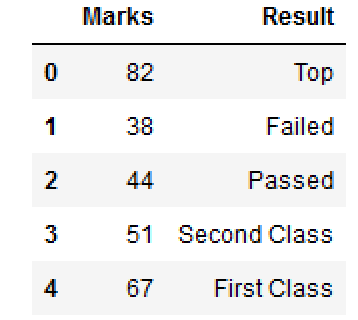Net-informations.com

# New dataframe column based on a given condition

There are times when you would like to add a new DataFrame column based on some condition . Actually, there does not exist any Pandas library function to achieve this method directly.

Suppose you have a DataFrame like this:

You want to create a new column "Result" based on the following condition:

1. A == B: 0
2. A > B: 1
3. A < B: -1

So, by applying above condition, DataFrame should be:

How yo can achieve above condition through Pandas DataFrame operation?

Lets create a DataFrame..

## Vectorized Version

#### Full Source## Operation on Single column

Suppose, you have a DataFrame like this:

You would like to add one more column for Result based on certain conditions.

1. Marks <= 30 : Failed
2. Marks >= 40 and <=49 : Passed
3. Marks >= 50 and <=59 : Second Class
4. Marks >= 60 and <=79 : First Class
5. Marks >= 80 and <=100 : Top

How you can create a dataFrame column based on the above condition using DataFrame.loc[] .

#### Full Sourcenet-informations.com (C) 2022    Founded by raps mk